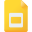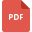# decimal and fractions tutorialsIntroduction to Decimals
Mathematics, Grade 7, United States, Decimal and FractionsWorksheet of Fractions and Decimals
Fraction, Grade 3, United States, Decimal and Fractions, MathematicsMultiplying and Dividing Decimals
Mathematics, Grade 7, United States, Decimals and Division, Decimals and Multiplication, Decimal and FractionsDecimals and Fraction Equivalent for Tenths
Decimal and Fractions, Grade 4, United States, MathematicsOrdering Numbers with up to 3 Decimal Places
Decimal and Fractions, Grade 4, United States, MathematicsDecimals to Percentages
Mathematics, Grade 7, United States, Decimal and Fractions, Percentages